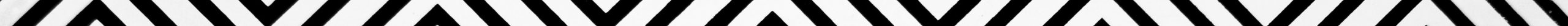There are four main math properties on operations of numbers. They are:

• Closure property
• Commutative property
• Associative property
• Distributive property.

The four math properties are defined on the four major arithmetic operations of addition, subtraction, multiplication and division. Apart from the four main math properties, there are individual math properties specific to the math operation such as multiplication and addition. Below, we will discuss the four math properties on the four arithmetic operations on the set of real numbers. Now, real numbers include integers and non-integers. Non-integers encompass rational and irrational numbers. Addition obeys closure, commutative, associative properties among the four math properties.Addition of numbers possesses two more specific properties. They are

Distributive property is defined on addition in conjunction with multiplication. Let us now demonstrate how addition obeys the math properties as below:### 1. Closure property:

The sum of two real numbers is again another real number.
Eg: 2 + 3 = 5.

### 2. Commutative property:

The arithmetic operation of addition follows commutative property because the sum of numbers is same in whichever order the operation may be carried out.
Simply put, addition of numbers yields a same sum in whatever order they may be added.

Eg: 2 + 3 = 3 + 2 = 5